## Bayesian linear and nonlinear DSGE models

The bayes prefix now supports the commands dsge and dsgenl to fit Bayesian linear and nonlinear dynamic stochastic general equilibrium (DSGE) models.

DSGE models are popular in macroeconomics and finance to model multiple time series. The models contain systems of linear or nonlinear equations that describe various economic processes. The parameters of DSGE models typically have direct interpretation in the context of economic theory and often have established ranges of plausible values. These ranges (or constraints) should be incorporated in the estimation to obtain stable results.

Bayesian estimation provides a natural way to incorporate such constraints by specifying various prior distributions for model parameters that satisfy the constraints.

## Linear DSGE model

A DSGE model consists of a system of equations that relate economic variables, like inflation, the interest rate, and the output gap, to each other and to exogenous state variables that drive the system. Equations can be dynamic in two senses: lags capture backward-looking behavior, while expected future values capture forward-looking behavior. DSGE models can be used to analyze policies, like the effect of monetary shocks, fiscal shocks, etc.

Bayesian estimation consists of two steps: specifying the DSGE model and specifying priors for the DSGE model parameters.

A small New Keynesian model has an investment–saving (IS) equation that links the output gap to the interest rate, a Phillips curve that links inflation to the output gap, and a Taylor rule that links the interest rate to inflation. In Stata syntax, the model looks like this: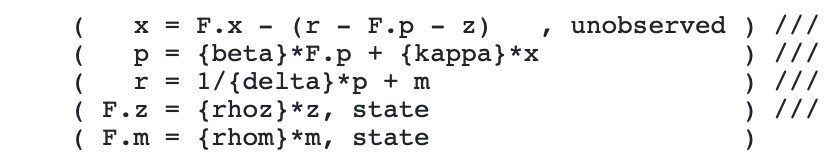The first equation is an IS curve, the second is a Phillips curve, and the third is a Taylor rule. The fourth equation is an IS shock, and the fifth equation is a Taylor rule monetary shock.

The unobserved option in the IS curve indicates that we treat the output gap as unobserved. We fit the model to data on inflation and the interest rate.

We add a prior specification for each parameter. Many DSGE parameters have interpretations that naturally restrict the range of admissible values. Parameter {beta} in the Phillips curve is a discount rate and must lie between 0 and 1. Parameter {kappa} is a price adjustment parameter, often thought to be small but positive. Parameter {delta} is the inverse of the Taylor rule inflation adjustment coefficient, so it must lie between 0 and 1. In addition to these range restrictions, one might have priors on the probable location of the parameter value. The discount rate is probably around 0.95, the inflation adjustment parameter around 0.2, and the inverse inflation adjustment coefficient around 0.66 (for a Taylor rule coefficient of 1.5). Priors allow you to incorporate that information explicitly in estimation.

Consider the data on the inflation rate and federal funds rate since 1955.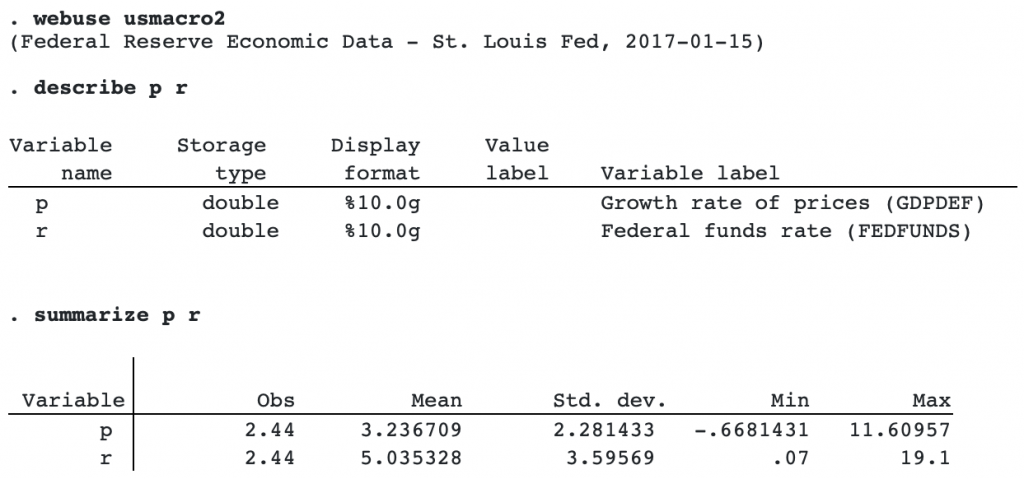Gathering up both the prior specification and the model specification, our estimation command is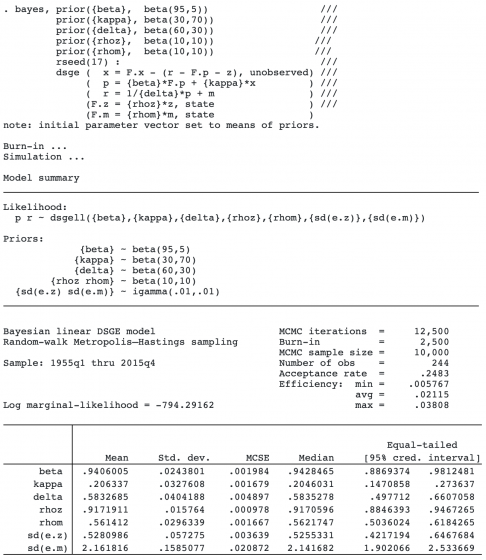The model summary shows the likelihood model and the prior specifications. The priors for all but the standard deviations are required. In the case of DSGE models, this makes sense because parameters are related to economic theory. For example, the prior for the discount factor {beta} is beta with shape parameters 95 and 5. The standard deviations of the shocks to the state variables z and m were assigned default inverse gamma priors.

The estimation output displays the number of burn-in periods (2,500), the MCMC sample size (10,000), the sample size of the data (244 periods), the sample period (1955q1 through 2015q4), and the acceptance rate and efficiency of the estimation process. The acceptance rate refers to the fraction of MCMC draws that was accepted. Typical values for the Metropolis–Hastings sampler used are 20% to 30%; our acceptance rate is 24%. Efficiency is related to the autocorrelation of the MCMC draws and indicates how quickly the sampler traverses the parameter space. Our average efficiency is 2.1%.

The estimation output displays posterior parameter means, standard deviations, medians, and equal-tailed credible intervals. The posterior mean of {beta} is 0.94, very close to its prior mean of 0.95. The posterior mean of {kappa} is about 0.21, lower than the prior mean of 0.30. The posterior mean for {delta} is 0.58, lower than the prior mean of 0.66. Both shocks are highly persistent, with posterior mean autocorrelation coefficients on z and m of 0.92 and 0.56, respectively. The posterior means of the standard deviations of the shocks are 0.53 for the IS shock z and 2.16 for the Taylor rule monetary shock m.

All of Stata’s Bayesian postestimation features work after bayes: dsge.

We can plot the posterior density of the prior adjustment parameter {kappa}.

```. bayesgraph kdensity {kappa}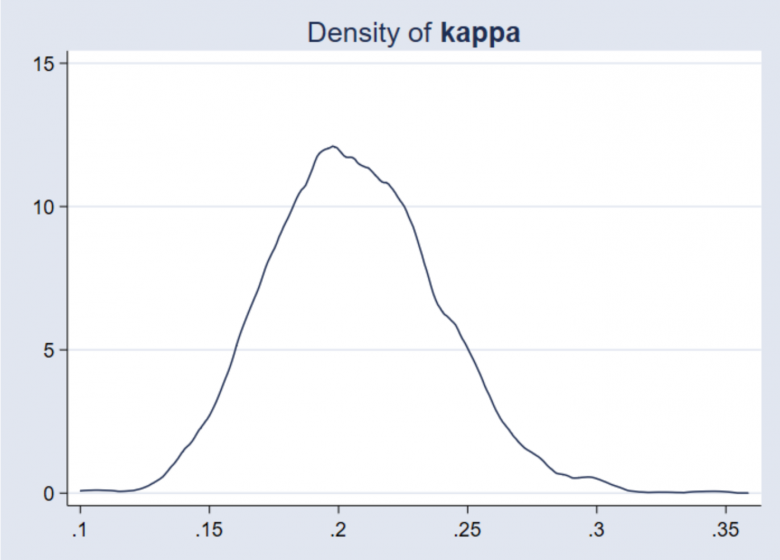```

We can plot a full set of diagnostics for {kappa}.

```. bayesgraph diagnostics {kappa}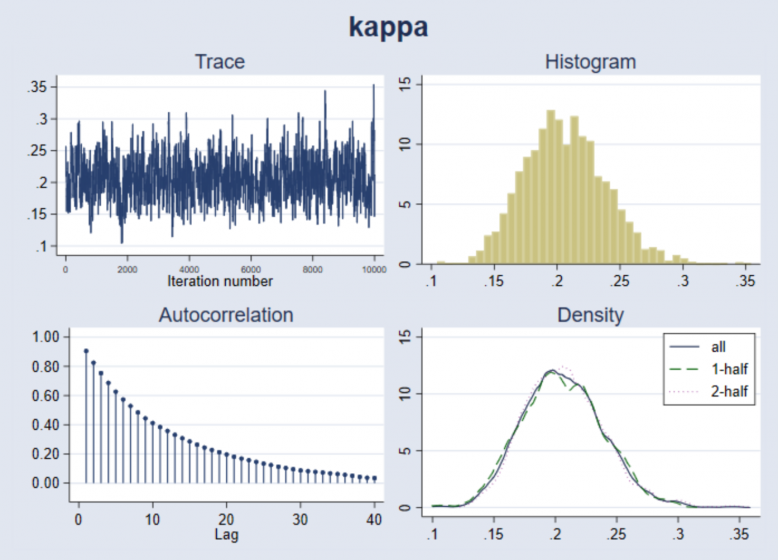```

We can add the prior density to the posterior density plot to visually compare the prior and posterior.

```. bayesgraph kdensity kappa, addplot(function Prior = betaden(30,70,x), ///
legend(on label(1 "Posterior")) lcolor(blue))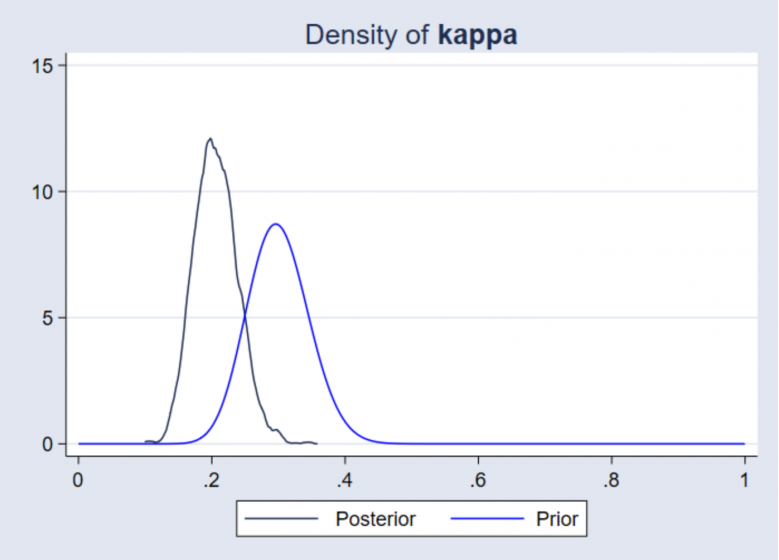We can examine posterior densities of functions of model parameters, such as the Taylor rule coefficient 1/{delta}.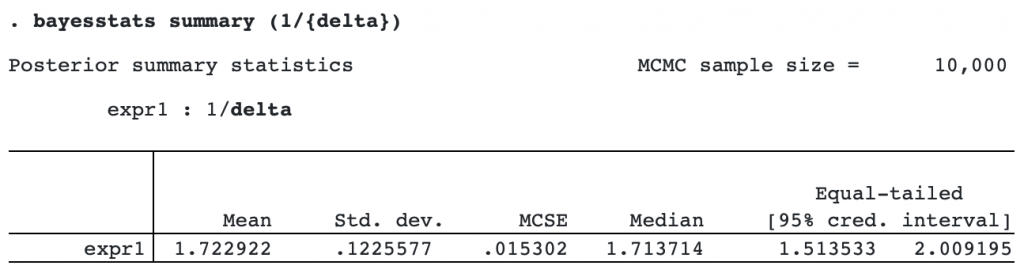```

The posterior mean for 1/{delta} is 1.7, higher than the prior mean of 1.5 and indicating a strong response of interest rates to changes in inflation.

We can compute Bayesian impulse–response functions. First, we save the MCMC results to a dataset, nk_mcmc.dta. We can do this during estimation with the saving() option or even after estimation by replaying the bayes command with the saving() option.

```. bayes, saving(nk_mcmc.dta, replace)
note: file nk_mcmc.dta not found; file saved.
```

With the MCMC sample saved, we can construct impulse–response functions with the bayesirf suite. This suite of commands extends irf to accommodate Bayesian estimation and is available after bayes: dsge, bayes: dsgenl, and bayes: var.

```. bayesirf set bayes_irfs.irf, replace
(file bayes_irfs.irf created)
(file bayes_irfs.irf now active)

. bayesirf create nk_model
(file bayes_irfs.irf updated)

. bayesirf graph irf, impulse(m) response(x p r m)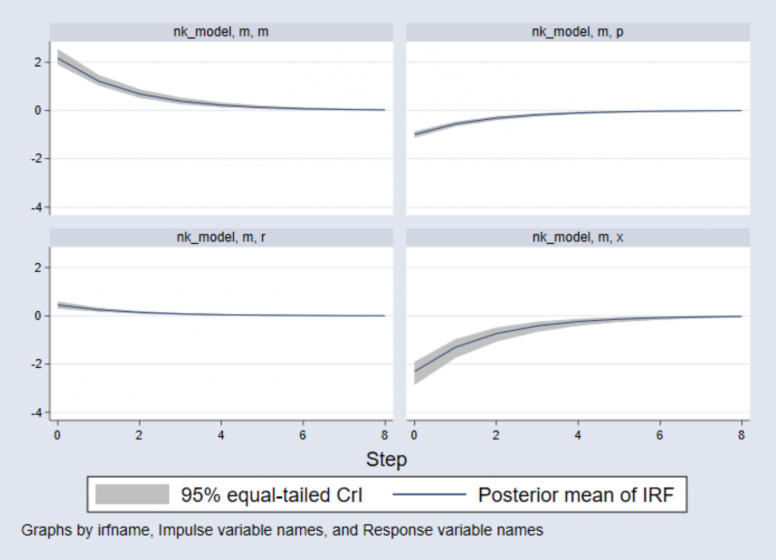```

The top-left panel shows the response of the monetary state variable m to a shock to itself. The top-right panel shows the response of inflation to the shock: it declines then returns monotonically to steady-state. The bottom-left panel shows the response of the interest rate: it rises. Finally, the bottom-right panel shows the response of the output gap: it falls below 0, indicating a recession. The contractionary monetary shock thus raises the interest rate, reduces inflation, and causes output to decline.

All this and more can be performed with the bayes: dsge command.

## Nonlinear DSGE model

The bayes prefix also works with the dsgenl command, which estimates the parameters of nonlinear DSGE models by first-order perturbation.

Next we estimate the parameters of a stochastic growth model. The model has equations for consumption, the real interest rate, GDP, the capital stock, and productivity.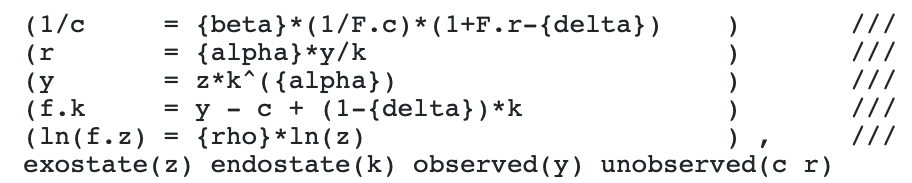Macroeconomists will recognize these relationships as a consumption Euler equation, a capital demand equation, a production function, a capital accumulation equation, and a law of motion for productivity, respectively.

The parameters have interpretation: {beta} is the discount rate, usually thought to be around 0.95; {alpha} is capital’s share of income, usually thought to be about one-third; {delta} is the depreciation rate, usually 2.5% per quarter; and {rho} is the persistence of the technology shock. Priors are used to bring this information to bear on the estimation.

We estimate the parameters of this model using detrended output. The m1gdp.dta dataset provides the logarithm of output.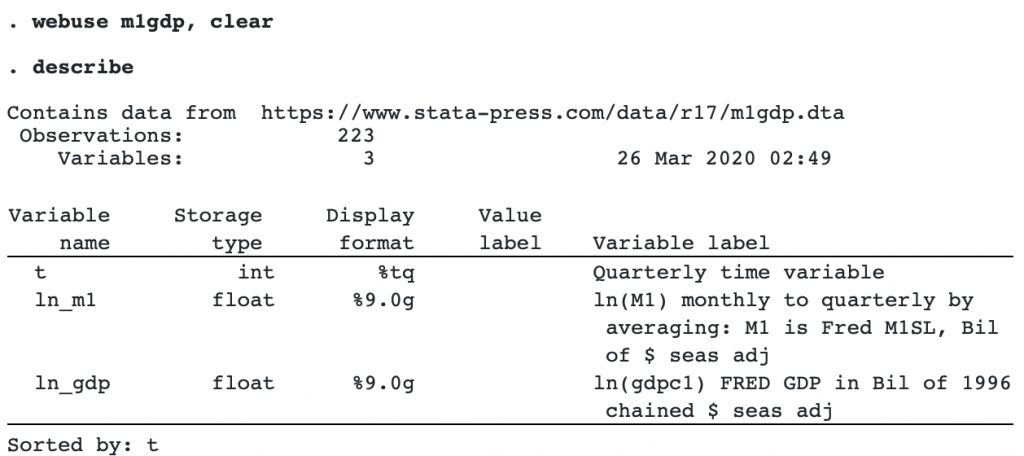The tsfilter command extracts the trend component of output. We use a Hodrick–Prescott filter and then scale the result up by a factor of 100. The resulting scale is such that a value of 1 indicates that GDP is 1% above its trend value.

```. tsfilter hp y = ln_gdp

. replace y = 100*y
(222 real changes made)

. tsline y, title("HP-detrended output")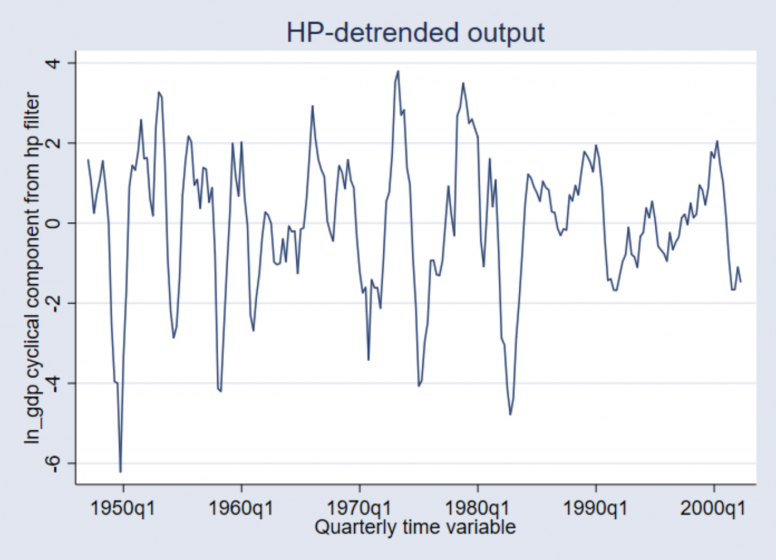```

Recessions are associated with a decline in output below trend, while booms are associated with a rise in output above trend.

We proceed with parameter estimation.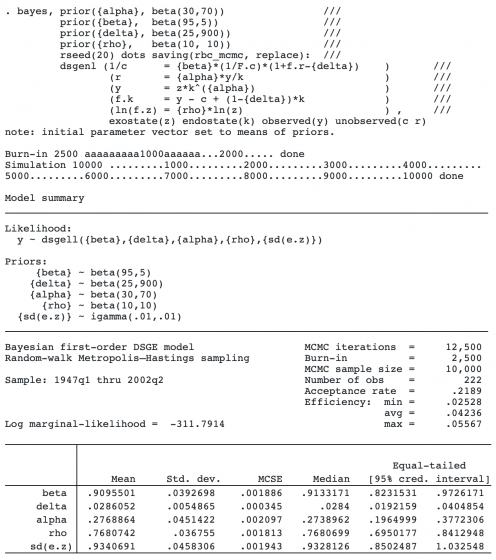The prior mean of the discount rate {beta} is 0.95; its posterior mean is little changed at 0.91. The prior mean of the depreciation rate {delta} is 0.025; its posterior mean is little changed at 0.0286. The prior mean of {alpha} is 0.3; its posterior mean is slightly lower at 0.28. Meanwhile, the posterior mean of {rho} is 0.77, much larger than its prior mean of 0.5. The standard deviation of the technology shock is about 1.

We next compute impulse–response functions to a technology shock.

```. bayesirf set bayes_irf2.irf, replace
(file bayes_irf2.irf created)
(file bayes_irf2.irf now active)

. bayesirf create rbc_model, step(16)
(file bayes_irf2.irf updated)

. bayesirf graph irf, impulse(z) byopts(yrescale)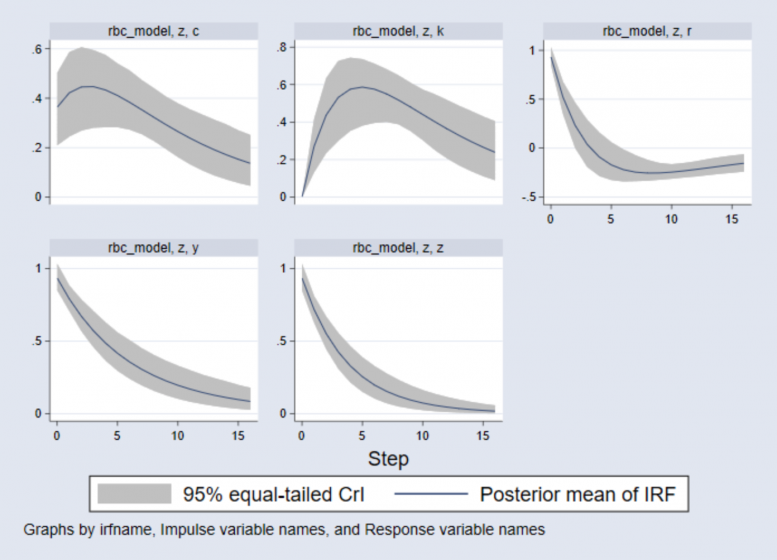```

The vertical axis units are in percentage deviations from steady-state. The horizontal axis units are in the same time duration as the data, that is, quarters. We plot 16 steps (4 years) of responses.

• The consumption response is plotted in the top-left panel. Consumption rises on impact and then continues to rise in a hump-shaped pattern before falling back to its steady-state.
• The capital stock response is plotted in the top-middle panel. The capital stock does not move on impact but afterward rises to a peak of 0.6% above its steady-state before declining.
• In the top-right panel, the interest rate rises on impact and then falls; it dips below 0 after four quarters and then returns to its steady-state from below.
• The bottom-left panel plots the response of output; it rises on impact and then falls monotonically back to its steady-state.
• The bottom-middle panel plots the response of technology itself. It, too, rises on impact and then falls monotonically back to its steady-state.

Exact posterior means and credible intervals can be viewed with bayesirf table. Below, we tabulate the response of consumption to a technology shock.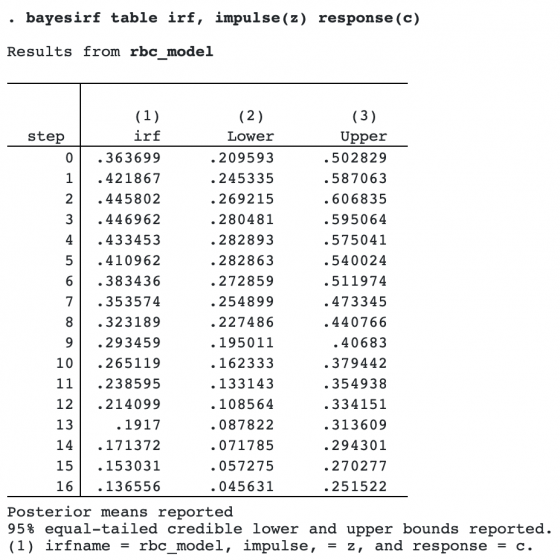After a one-standard-deviation technology shock, consumption rises and peaks at 0.447% above its steady-state value in the third period after the shock.